# Polygon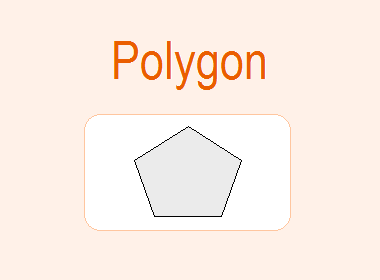A closed bounded geometrical figure with three or more sides is called a polygon. There are specific names of the polygon according to the number of sides in the polygon. They are as follows: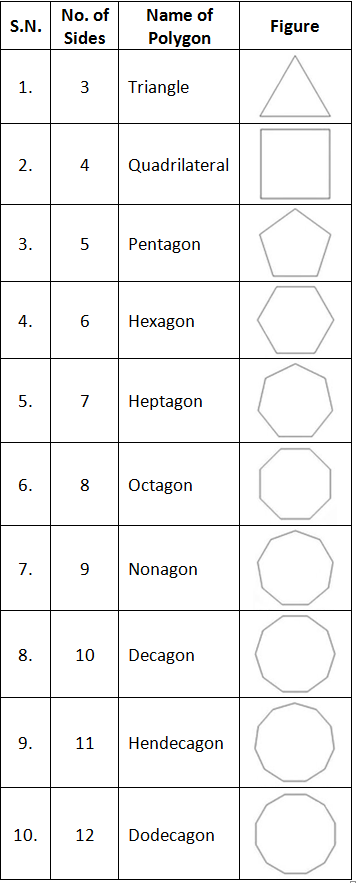********************## Interior and Exterior Angle of a Polygon

An angle that forms inside the polygon is called an interior angle. When a side of a polygon is produced, the angle that forms outside of the polygon is called an exterior angle.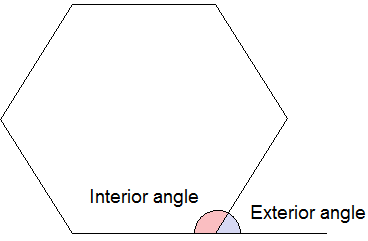The sum of interior angles of a polygon is given by the formula (n – 2) × 180° where n is the number of sides of the polygon.

i.e. Sum of interior angles of a polygon = (n – 2) × 180°

Therefore, by using this formula,

Sum of interior angles of a triangle = 180°

Sum of interior angles of a quadrilateral = 360°

Sum of interior angles of a pentagon = 540°

Sum of interior angles of a hexagon = 720°

Sum of interior angles of a heptagon = 900°

Sum of interior angles of an octagon = 1080°

Sum of interior angles of a nonagon = 1260°

Sum of interior angles of a decagon = 1440°

Sum of interior angles of a hendecagon = 1620°

Sum of interior angles of a dodecagon = 1800°

## Regular Polygon

A polygon having all the sides equal and each interior angle also equal is called a regular polygon. The Interior and exterior angles of a regular polygon is given by the formula,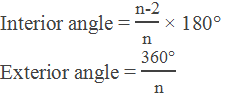## Construction of Regular Polygon

Example 1: Construct a regular pentagon of side 4 cm.

Solution: Here,

Length of side = 4 cm

No. of side (n) = 5

Interior angle = (n – 2)180°/n

= (5 – 2)180°/5

= 108°

### Steps of construction:

Step 1: Draw a baseline XY and cut an arc of 4 cm on the line XY and mark it AB.

Step 2: Draw 108° angle at points A and B using a protractor.

Step 3: Cut 4 cm arc AF and BG and mark as E and C.

Step 4: Make an angle of 108° at points E and C.

Step 5: Draw EH and CF and mark point D at the cross point.Hence, ABCDE is a regular pentagon of side 4 cm.

Example 2: Construct a regular hexagon of side 3 cm.

Solution: Here,

Length of side = 3 cm

No. of side (n) = 6

Interior angle = (n – 2)180°/n

= (6 – 2)180°/6

= 120°

### Steps of construction:

Step 1: Draw a baseline XY and cut an arc AB of 3 cm on XY.

Step 2: Make an angle 120° at each vertex and cut an arc of 3 cm in each. Join the line segment.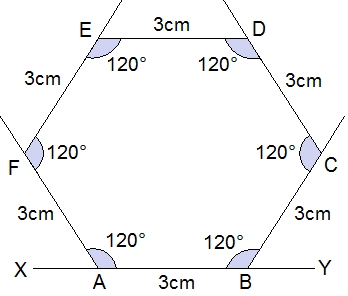Hence, ABCDEF is a regular hexagon of side 3 cm.

Example 3: Construct a regular octagon of side 3.5 cm.

Solution: Here,

Length of side = 3.5 cm

No. of side (n) = 8

Interior angle = (n – 2)180°/n

= (8 – 2)180°/8

= 135°

### Steps of construction:

Step 1: Draw a baseline XY and cut an arc AB of 3.5 cm on XY.

Step 2: Make an angle 135° at each vertex and cut an arc of 3.5 cm in each. Join the line segment.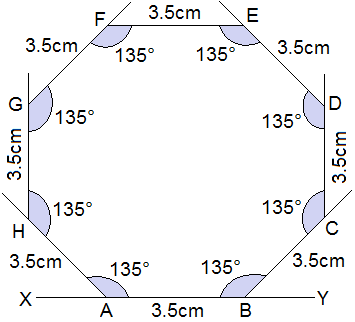Hence, ABCDEFGH is a regular octagon of side 3.5 cm.

## Worked Out Examples

Example 4: Find the value of x.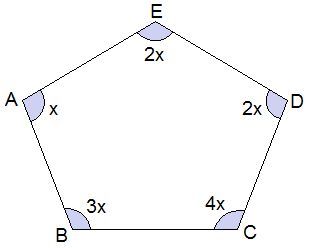Solution: Here,

From the figure,

No. of sides (n) = 5

Sum of interior angles = (n – 2)180°

i.e. 3x + 4x + 2x + 2x + x = (5 – 2)180°

or, 12x = 540°

or, x = 540°/12

or, x = 45° Ans.

Example 5: Find the value of x.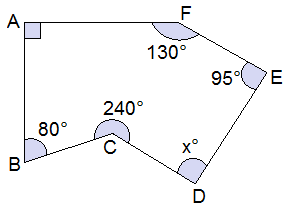Solution: Here,

From the figure,

No. of sides (n) = 6

Sum of interior angles = (n – 2)180°

i.e. A + B + C + D + E + F = (6 – 2)180°

or, 90° + 80° + 240° + x + 95° + 130° = 720°

or, x + 635°  = 720°

or, x = 720° - 635°

or, x = 85° Ans.

Example 6: Find the number of sides of a regular polygon where each interior angle is 120°.

Solution: Here,

Each interior angle = 120°

i.e. (n – 2)180°/n = 120°

or, (n – 2)180° = 120°n

or, 180°n - 360° = 120°n

or, 180°n - 120°n = 360°

or, 60°n = 360°

or, n = 360°/60°

or, n = 6

Number of side (n) = 6 Ans.